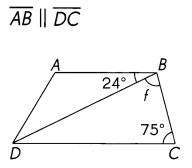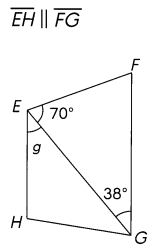# Math in Focus Grade 5 Chapter 13 Practice 5 Answer Key Parallelogram, Rhombus, and Trapezoid

This handy Math in Focus Grade 5 Workbook Answer Key Chapter 13 Practice 5 Parallelogram, Rhombus, and Trapezoid provides detailed solutions for the textbook questions.

## Math in Focus Grade 5 Chapter 13 Practice 5 Answer Key Parallelogram, Rhombus, and Trapezoid

Complete. Figure ABCD is a parallelogram. Measure the sides and angles of the figure.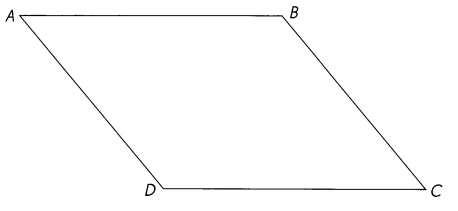Explanation: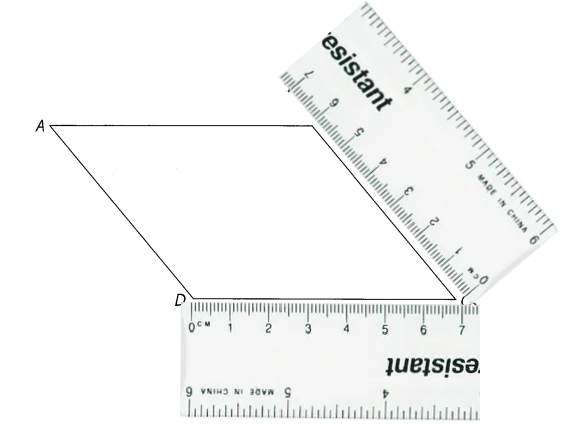Question 1.
Explanation:
Property of a Parallelogram:

• Opposite sides are equal and parallel.

Question 2.
AB _______ cm
AB = 7 cm
Explanation:
Property of a Parallelogram:

• Opposite sides are equal and parallel.

Question 3.
BC = __ cm
BC = 6 cm
Explanation:
Property of a Parallelogram:

• Opposite sides are equal and parallel.

Question 4.
DC = ______ cm
DC = 7 cm
Explanation:
Property of a Parallelogram:

• Opposite sides are equal and parallel.

Question 5.
m∠A = ____
m∠A = 55°
Explanation:
Property of a Parallelogram:

• Opposite angles are equal.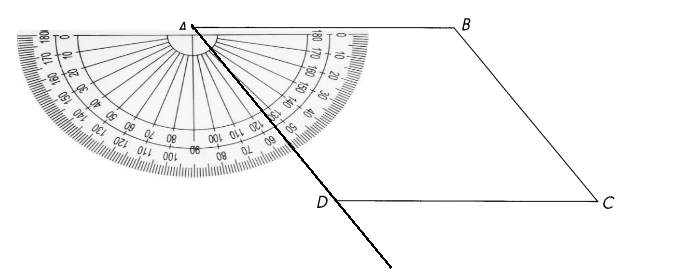Question 6.
m∠B = ___
m∠B = 120°

Explanation:
Property of a Parallelogram:

• Opposite angles are equal.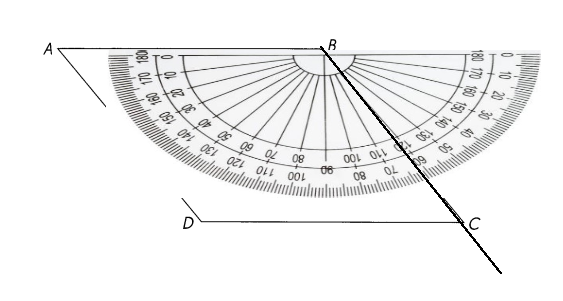Question 7.
m∠C = ___
m∠C = 55°

Explanation:
Property of a Parallelogram:

• Opposite angles are equal.

Question 8.
m∠D = ___
m∠D = 120°

Explanation:
Property of a Parallelogram:

• Opposite angles are equal.

Question 9.
Name the parallel sides of the figure. ______
$$\overline{A B}$$ || $$\overline{D C}$$
$$\overline{A D}$$ || $$\overline{B C}$$

Question 10.
Name the opposite angles that are equal. ______
m∠A = m∠C
m∠B = m∠D

This parallelogram is not drawn to scale. Fill in the blanks.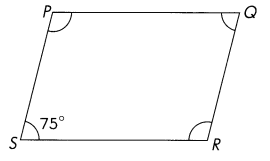Question 11.
m∠Q = m∠___
= _____
m∠Q = m∠S = 75°

Explanation:
Property of a Parallelogram:

• Opposite angles are equal.

Question 12.
m∠P = 180° – ___
= ____
m∠P = 180° – m∠S =180° – 75° = 105°

Explanation:
Property of a Parallelogram:

• Sum of any two adjacent angles is 180°

Question 13.
m∠R = m∠___
= ____
m∠R = m∠P =  105°

Explanation:
Property of a Parallelogram:

• Opposite angles are equal.

These parallelograms are not drawn to scale. Find the unknown angle measures.

Question 14.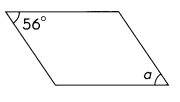m∠a =  56°
Explanation:
Property of a Parallelogram:

• Opposite angles are equal.
m∠a =  56°

Question 15.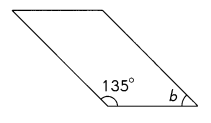m∠b = 45°

Explanation:
Property of a Parallelogram:

• Sum of any two adjacent angles is 180°
m∠b = 180-135 =45°

Question 16.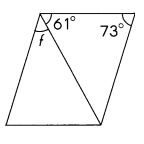m∠f = 46°

Explanation:
Property of a Parallelogram:

• Sum of any two adjacent angles is 180°
180-73=107°
m∠f = 107 – 61 =46°

Question 17.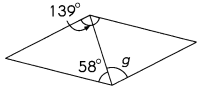m∠g =  81°
Explanation:
Property of a Parallelogram:

• Opposite angles are equal.
So opposite angle is 139°
m∠g =  139 -58 = 81°

Question 18.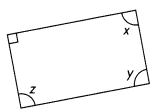m∠x = 90°
m∠y = 90°
m∠z = 90°

Property of a Parallelogram:

• Opposite angles are equal.
• Sum of any two adjacent angles is 180°
m∠y = 90°
m∠x = 180 -90° =90°
m∠z = m∠x = 90°

Question 19.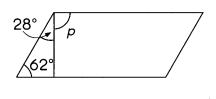m∠p = 90°

Property of a Parallelogram:

• Sum of any two adjacent angles is 180°
m∠p = 180-62+28= 90°

Complete. Write the name of another side or angle of each rhombus.

Question 20.
AB = BC
= ___ = ____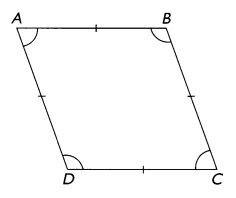AB = BC= CD= DA
Explanation:
property of a Rhombus:

• All sides are equal and, opposite sides are parallel to each other.
AB = BC= CD= DA

Question 21.
m∠B = m∠______
m∠B = m∠D
Explanation:
property of a Rhombus:

• Opposite angles are equal.
m∠B = m∠D

Question 22.
m∠A = m∠____
m∠A = m∠C
Explanation:
property of a Rhombus:

• Opposite angles are equal.
m∠A = m∠C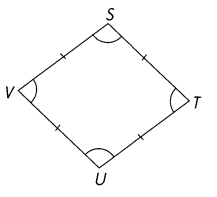Question 23.
UV = _______
= ___ = ___
UV = VS= ST = TU

Explanation:
property of a Rhombus:

• All sides are equal and, opposite sides are parallel to each other.
UV = VS= ST = TU

Question 24.
m∠S = m∠____m∠S = m∠U

Explanation:
property of a Rhombus:

• Opposite angles are equal.
m∠S = m∠U

Question 25.
m∠T = m∠______
m∠T = m∠V

Explanation:
property of a Rhombus:

• Opposite angles are equal.
m∠T = m∠V

This rhombus is not drawn to scale. Fill in the blanks.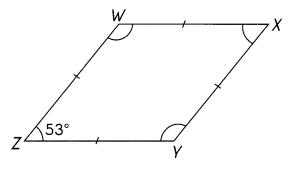Question 26.
m∠X = m∠__ = ____
m∠X = m∠Z= 53°

Explanation:
property of a Rhombus:

• Opposite angles are equal.
m∠X = m∠Z= 53°

Question 27.
m∠W = ____ – ___ = ____
m∠W = 180 – 53° = 127°

Explanation:
property of a Rhombus:

• Sum of any two adjacent angles is 180°
m∠W = 180 – m∠Z = 180 – 53° = 127°

Question 28.
m∠Y = m∠___ = ____
m∠Y = m∠W = 127°

Explanation:
property of a Rhombus:

• Opposite angles are equal.
m∠Y = m∠W = 127°

These rhombuses are not drawn to scale. Find the unknown angle measures.

Question 29.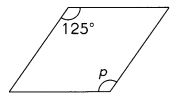m∠p =  125°

Explanation:
property of a Rhombus:

• Opposite angles are equal.
m∠p= 125°

Question 30.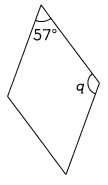m∠q = 180 – 57° = 123°

Explanation:
property of a Rhombus:

• Sum of any two adjacent angles is 180°
m∠q = 180 – 57° = 123°

Question 31.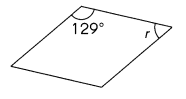m∠r = 180 – 129° = 51°

Explanation:
property of a Rhombus:

• Sum of any two adjacent angles is 180°
m∠r = 180 – 129° = 51°

Question 32.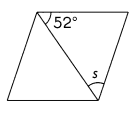m∠s =  52°

Explanation: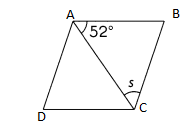property of a Rhombus:
• All sides are equal and, opposite sides are parallel to each other.
AB=BC=CD=DA

then in Triangle ABC AB= BC. then it is isosceles Triangle .
The isosceles triangle property states that when two sides are equal, the base angles are also equal.
So, m∠s =  52°

Question 33.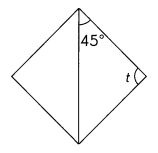m∠t =  90°

Explanation: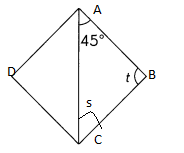property of a Rhombus:
• All sides are equal and, opposite sides are parallel to each other.
AB=BC=CD=DA

then in Triangle ABC AB= BC. then it is isosceles Triangle .
The isosceles triangle property states that when two sides are equal, the base angles are also equal.
So, m∠s =  45°
in triangle sum of three angles is 180°
So, m∠t = 180 -45+  45° = 90°

Question 34.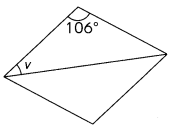m∠s =  37°

Explanation: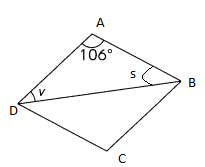in triangle ABC  sum of three angles is 180°
m∠s  + m∠v = 180°- 106°=  74°

property of a Rhombus:
• All sides are equal and, opposite sides are parallel to each other.
AB=BC=CD=DA

then in Triangle ABC AB= BC. then it is isosceles Triangle .
The isosceles triangle property states that when two sides are equal, the base angles are also equal.
So, m∠s =m∠v  =  74° divided by 2 = 37°

Measure the unknown angles. Then fill in the blanks. ABCD is a trapezoid where $$\overline{A B}$$ || $$\overline{D C}$$.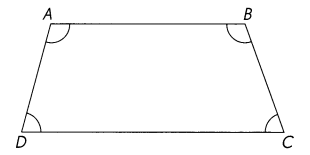Question 35.
m∠A = ____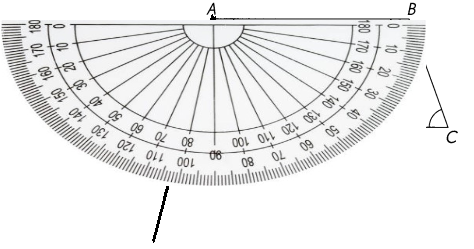Question 36.
m∠B = ___
m∠B = 110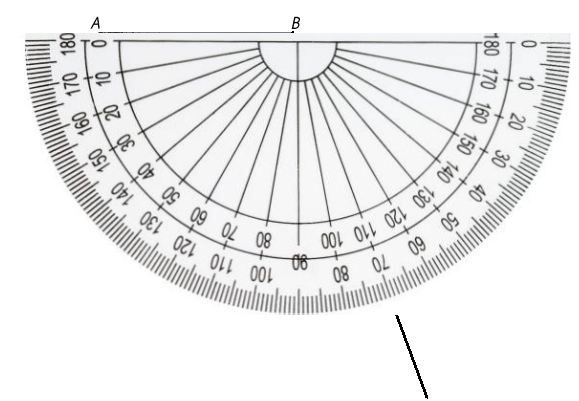Question 37.
m∠C = ____
m∠C = 70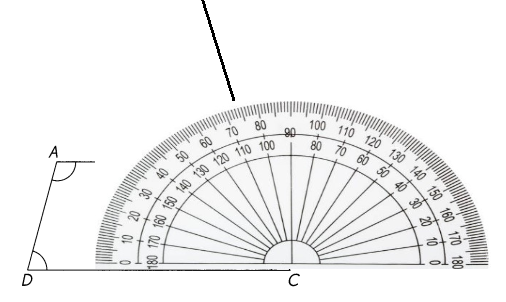Question 38.
m∠D = ___
m∠D = 80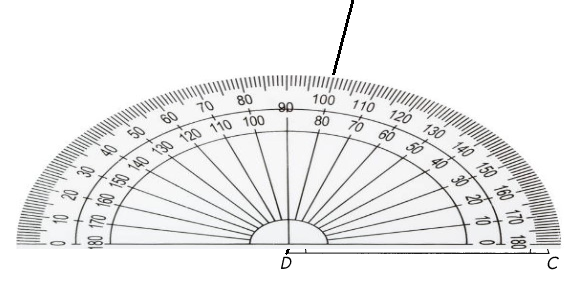Question 39.
m∠A + m∠D = m∠___ + m∠___ = ____
m∠A + m∠D = m∠100+ m∠80= 180
Supplementary angles = 180°

These trapezoids are not drawn to scale. Find the unknown angle measures.

Question 40.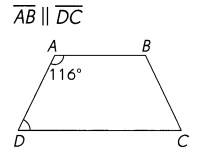m∠A  = m∠116°
m∠D = m∠64°
m∠D  = 180 – 116
= 64°
Explanation:
Supplementary angles = 180°
m∠64° + m∠116° = 180°

Trapezium and Its Properties
• Angle: The sum of angles in a trapezoid-like other quadrilateral is 360°. …
• Two angles on the same side are supplementary, that is the sum of the angles of two adjacent sides is equal to 180°.

Question 41.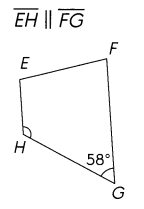m∠G = m∠58°
m∠F = m∠122°
m∠F  = 180 – 58 = 122
Explanation:
Supplementary angles = 180°
m∠58° + m∠122° = 180°

Question 42.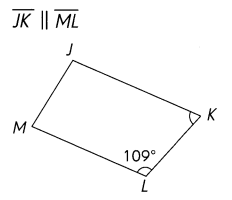m∠L = m∠109°
m∠K = m∠71°
m∠K  = 180 – 109 = 71
Explanation:
Supplementary angles = 180°
m∠71° + m∠109° = 180°

Question 43.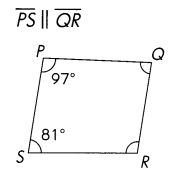m∠P = m∠97°
m∠Q = m∠83°
m∠Q  = 180 – 97 = 83°
Explanation:
Supplementary angles = 180°
m∠83° + m∠97° = 180°
Explanation:
m∠S = m∠81°
m∠R = m∠99°
m∠R  = 180 – 81 = 99°
Explanation:
Supplementary angles = 180°
m∠81° + m∠99° = 180°

These trapezoids are not drawn to scale. Find the unknown angle measures.

Question 44.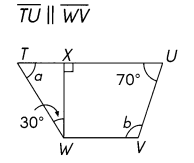m∠a = 60°
Explanation:
m∠w = 30°
m∠x = 90
90 + 30 = 120
m∠a = 180 – 120 = 60°
Sum of the angles of a triangle = 180°
m∠b = 110°
m∠u = 70° given
m∠u + m∠b  = 180°
180 – 70 = 110°
110 + 70 = 180°
Supplementary angles = 180°

Question 45.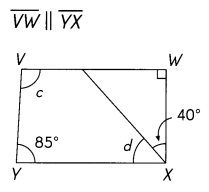∠d = 55°
∠c = 90°
Explanation:
In quadrilateral the sum of angles = 360°
∠w = 90°
∠Y = 85°
YX = 180°
85° + 40° = 125°
180° – 125° = 55°
∠d = 55°
125° + 55° + 90° = 270°
360° – 270° = 90°
∠c = 90°

Question 46.